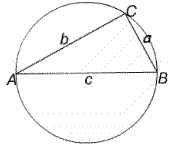You may also likeFixing It

A and B are two fixed points on a circle and RS is a variable diamater. What is the locus of the intersection P of AR and BS?OK! Now Prove It

Make a conjecture about the sum of the squares of the odd positive integers. Can you prove it?Summats Clear

Find the sum, f(n), of the first n terms of the sequence: 0, 1, 1, 2, 2, 3, 3........p, p, p +1, p + 1,..... Prove that f(a + b) - f(a - b) = ab.

Cyclic Triangles

Age 16 to 18Challenge LevelA triangle $ABC$ is inscribed in a circle with $AB$ as diameter. Find the maximum value of $AC + CB$.

Now generalise your result to the case where $AB$ is fixed but not a diameter of the circle.

Make and prove a conjecture about the cyclic quadrilateral inscribed in a circle of radius $r$ that has the maximum perimeter and the maximum area.